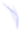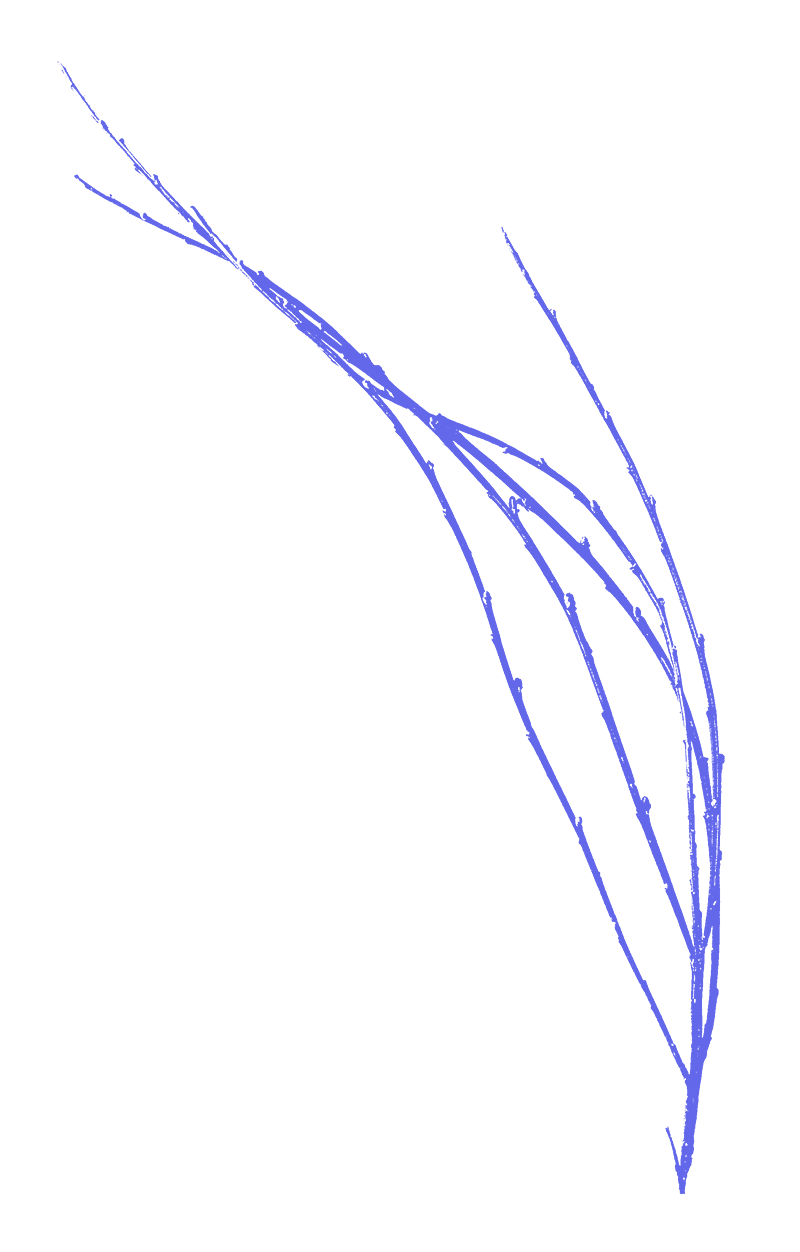## ((((((((((((((((((((((((((((((((((((((((((((((((((((((((((((((((((((((((((((((((((((((((((((((((((((((((((((((((((((((((((((((((((((((((((((((((((((((((((((((((((((((((((((((((((((((((((((((((((((((((Opportunities))))))))))))))))))))))))))))))))))))))))))))))))))))))))))))))))))))))))))))))))))))))))))))))))))))))))))))))))))))))))))))))))))))))))))))))))))))))))))))))))))))))))))))))))))))))))))))))))))))))))BOARC is an experiment in itself, which takes energy, good humour, self-reflection and lots of patience to run, and we thank you for your participation!

There are also opportunities for live-in stewards who can assist long term whilst using the space for their own projects, and affiliates - either individuals, groups or organisations - who can help steer the project over the next couple of years.

+
+
+
+